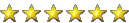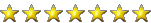# Fractal Generator FAQ and How-to

#### Oliver_FF

##### New Member
Ok, so how many of you have heard of a Fractal Generator? XD When I first created my own fractal generator from scratch I did some reading about it, and nothing was really laid out simply enough for me to fully understand it with really basic maths understanding, so i'm gonna attempt to correct thisWhat on earth is a fractal??
A fractal is an Infinitely Complex shape?
A little maths background
Sorry guys, a little maths has to come here. Suppose you have a quadratic equation (something that looks like this:

x^2 + 3x - 2 and you want to find it's "roots" (the places where the line crosses the X-axis. You --could-- solve this by solving the following X^2 +3x -2 = 0, but what if the equation is REALLY tough?

One way of doing this is to guess a number, and put it through the equation. Take the new result, put it in again. Take the new result and put it in again. Eventually your number will either run up to infinity or settle to one specific number. If it runs up and up, it's said to tend towards infinity.

The next part of maths you need to be aware of are "Imaginary Numbers"In particular, you need to know all about the number "i". i carries the value of squareroot(-1). Now, don't try to work out it's value, because it's impossible to squareroot a negative number and you'll just get errors on your calculators. The important thing about i is that i^2 = -1

A complex number in my context is something that looks like 3i + 5. It basically has two parts, a real number and an imaginary number.

So where's this going??
Well, a Fractal is drawn by using the method of solving a quadratic equation i mentioned earlier. You start by taking a massive grid, I've chosen to use 1024x768 pixels, as it's a cool numberYou then imagine a graphs axis running through the middle your image, as if your looking at some graph paper from a science experiment back at school.

Along the grid from left to right is the x-axis. The line from the top to the bottom is the i axis.

So what's a Fractal? It's a visual representation of which of your grid locations tend off towards infinity when you put them through any mathematical equation XD

The Mandelbrot Set
Click for a Preview!!!
This fractal is generated by using the equation x^2 + x. You take a co-ordinate in the form of a complex number, ie. for co-ordinate (1, 1) you have the complex number (1i + 1). You stick this number into the equation and you get a new number out. You put this new number into the equation and get a new number out... keep repeating. If the value you get out goes over say 4, then it's safe to assume that it has tended towards infinity. When this happens you can assign that specific co-ordinate a colour depending on how many times you've repeated the equation.

Using (1i + 1):
(1i + 1)^2 + (1i + 1) --> expand the first part
(1i + 1)(1i + 1) + (1i + 1) --> multiply out the bracket
(1i^2 + 2i + 1) + (1i + 1) --> simplify i^2 for -1
(-1 + 2i + 1) + (1i + 1) --> add the two together
(3i + 1)
the numbers 1 and 3 are both below 4, so we put (3i + 1) back into the formula.

Now, this isn't very easy to program, so i'll be nice and work out a scenario algebraically.
Suppose our co-ordinate is (ai + b) where a is the y co-ordinate and b is the x co-ordinate.

Putting that into the equation gives:
(ai + b)^2 + (ai + b)
(ai + b)(ai + b) + (ai + b)
((a^2)(i^2) + (2bai) + (b^2)) + (ai + b)
((-a^2) + 2bai + (b^2) + (ai + b)
b^2 + 2bai - a^2 + ai + b

Now, separate out the components involving i:
2bai + ai --> factor the i's out
(2ba + a)i
And those that don't:
b^2 - a^2 + b

Still with me? XD
For those that lost interest, here's the important bit
So, if you take this co-ordinate:
(ai + b)
then after you apply the formula you'll have this:
((2ba + a)i + (b^2 - a^2 + b))

The Julia Set
This is basically the same as the Mandelbrot set, but instead of having the equation x^2 + x, you use:
x^2 + c, where c is the initial co-ordinate, and always is.

The Julia set is said to be a Subset of the Mandelbrot set, because if you pick a point on in the mandelbrot fractal and put those values into the Julia set, you'll get something similar but different.

My Fractal Generator
Well, it seems i can't upload my app, but i suppose that's fair enough. I've written it using C++ and the SDL libraries in the Dev-C++ IDE, but i've found someone to host the app anyways.

You can see what it looks like in the screenshots below, you'll need the SDL.dll runtime file found here:
http://www.libsdl.org/release/SDL-1.2.12-win32.zip
just stick the DLL in the same directory as the .exe and it'll workAnd my app is here:

The background to the window is the Mandelbrot set, the top left window draws the Julia set with the corresponding constant relative to where your mouse is over the Mandelbrot set. The bottom left window draws the Mandelbrot set again, but you can move and zoom in infinitely to check out some of the cool features.

The Controls
Mouse cursor - alters the constant values for julia set and moves the zoom window.
Home/PageUp - increases the zoom in the zoom window.
End/PageDown - decreases the zoom in the zoom window.
Arrow Keys - fine tune the view in the zoom window.

#### Attachments

• 78.2 KB Views: 471
• 72.1 KB Views: 621
• 88.5 KB Views: 605
Last edited by a moderator:
•farlex85, Kreij and Solaris17

#### DrunkenMafia

#####Man I just woke up and this is the first thing I read....

Will try and read it again later on..#### Oliver_FF

##### New Member
long double a, b, c, e, f, g, h;

//void ColorPixel(x-pos, y-pos, R value, G value, B value); --> You'll need a way of plotting pixels!

//Mandelbrot set:
for (x=0; x<1024; x++){ //window size 1024x768
for (y=0; y<768; y++){
a = x-830; a = a/400; //Scale to fit the window!
b = y-400; b = b/320;
g=a;
h=b;
for (p = 0; p<255; p++){ //Loop some
c = a;
a = ((a * a) - (b * b)) + g;
b = (2 * c * b) + h;
if (a > 4) break;
if (b > 4) break;
}
PlotPixel(x,y,p*2,0,0); //Here's where you want to plot the pixel to screen!
}
}

 Gah for the forum removing my indenting!

#### Kreij

##### Senior Monkey Moderator
Nice article and program Oliver_FF

Use the [code][/code] tags to keep the indenting intact.

Last edited by a moderator:
•Oliver_FF

#### Cuzza

#####man i dont get it. probably why i failed first year calculus! passed it 2nd time round tho

#### Disparia

#####PHP/GD:

PHP:
``````\$imgX = 640;
\$imgY = 480;

\$img = imagecreatetruecolor(\$imgX, \$imgY);

for (\$x = 0; \$x < \$imgX; ++\$x) {
for (\$y = 0; \$y < \$imgY; ++\$y) {
\$a = \$x - 830;
\$a = \$a / 400;
\$b = \$y - 400;
\$b = \$b / 320;
\$g = \$a;
\$h = \$b;
for (\$p = 0; \$p < 255; ++\$p) {
\$c = \$a;
\$a = ((\$a * \$a) - (\$b * \$b)) + \$g;
\$b = (2 * \$c * \$b) + \$h;
if (\$a > 4 || \$b > 4) {
break;
}
}
imagesetpixel(\$img, \$x, \$y, imagecolorallocate(\$img, \$p, 0, 0));
}
}

imagejpeg(\$img);
imagedestroy(\$img);``````•Oliver_FF

#### Oliver_FF

##### New Member
Haha, nicely done - didn't think of that XD

Code:
``````        \$a = \$x - 830;
\$a = \$a / 400;
\$b = \$y - 400;
\$b = \$b / 320;``````
you'll be able to see more/pan the image/zoom in#### Oliver_FF

##### New Member
man i dont get it. probably why i failed first year calculus! passed it 2nd time round tho
Basically, it's a 2d graph of the time it takes for a co-ordinate to tend towards infinity for the equation y=x^2 + c

You get some truly weird shapes